# Texas Go Math Grade 6 Module 8 Answer Key Applying Ratios and Rates

Refer to our Texas Go Math Grade 6 Answer Key Pdf to score good marks in the exams. Test yourself by practicing the problems from Texas Go Math Grade 6 Module 8 Answer Key Applying Ratios and Rates.

## Texas Go Math Grade 6 Module 8 Answer Key Applying Ratios and Rates

Graph each point on the coordinate grid above.

Question 1.
B(9, 6)
The point B(9, 6) locate on an xy-coordinate graph, go nine units from the origin to the right to 6 on the horizontal axis and then, from that point. go 6 units up (using the y-axis scale).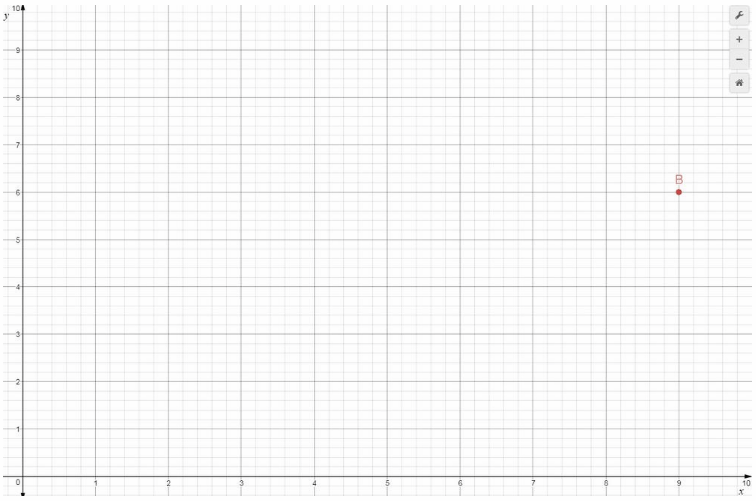Question 2.
C(0, 2)
The point C(0, 2) locate on an xy-coordinate graph, go zero units from the origin to 0 on the horizontal axis and then, from that point, go 2 units up (using the y-axis scale).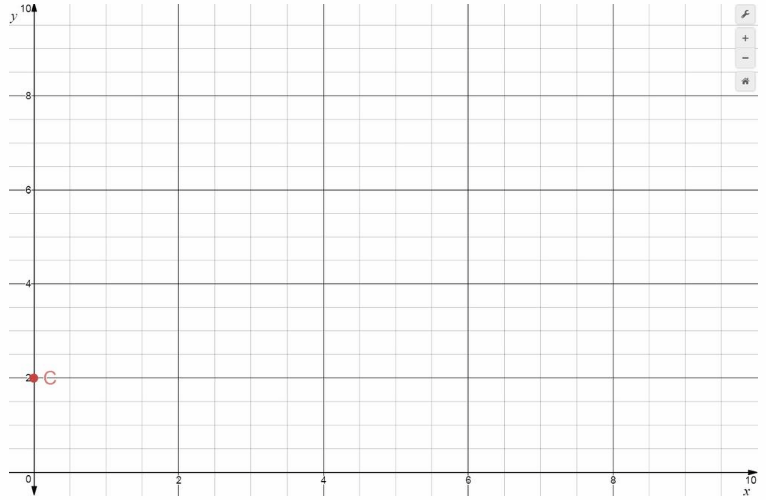Question 3.
D(6, 10)
The point D(6, 10) locate on an xy-coordinate graph, go six units from the origin to the right to 6 on the horizontal axis and then, from that point, go 10 units up (using the y-axis scale).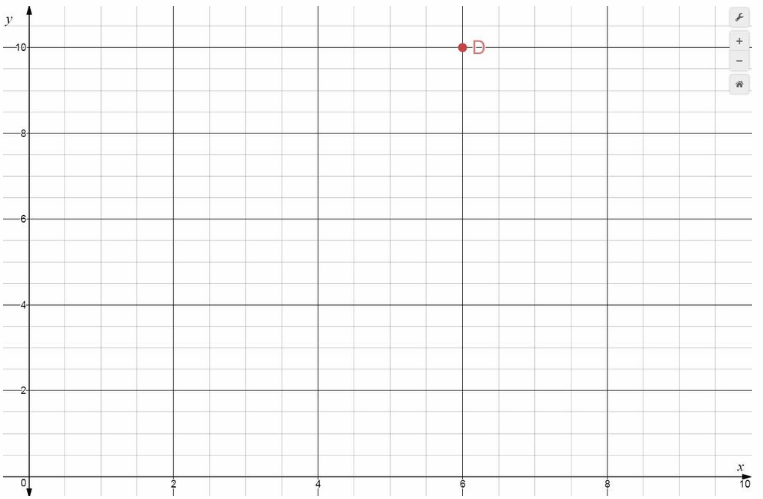Question 4.
E(3, 4)
The point E(3, 4) locate on an xy-coordinate graph, go three units from the origin to the right to 3 on the horizontal axis and then, from that point, go 4 units up (using the y-axis scale).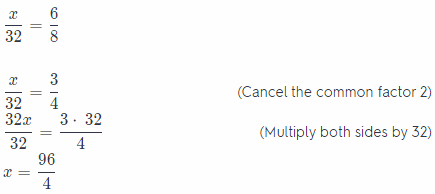Write the equivalent fractions.

Question 5.Switch sides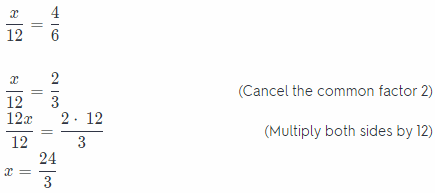x = 24

The equivalent fractions becomes
$$\frac{6}{8}=\frac{24}{32}$$

Question 6.Switch Sidesx = 7

The equivalent fractions becomes
$$\frac{1}{8}=\frac{7}{56}$$

Question 7.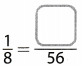Switch Sidesx = 7

The equivalent fractions becomes
$$\frac{1}{8}=\frac{7}{56}$$

Question 8.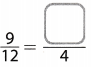Switch Sides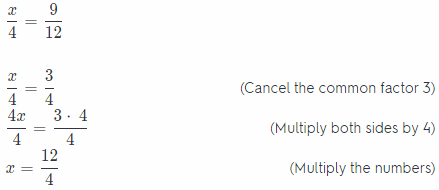x = 3

The equivalent fractions becomes
$$\frac{9}{12}=\frac{3}{4}$$

Question 9.First we use this rule
Apply fraction cross multiply: if $$\frac{a}{b}=\frac{c}{d}$$ then a ∙ d = b ∙ c
5x = 9 ∙ 25
5x = 225 (Multiply the numbers)
$$\frac{5 x}{5}=\frac{225}{5}$$ (Divide both sides by 5)
x = 25

The equivalent fraction becomes
$$\frac{5}{9}=\frac{25}{45}$$

Question 10.First we use this rule
Apply fraction cross multiply: if $$\frac{a}{b}=\frac{c}{d}$$ then a ∙ d = b ∙ c
5x = 6 ∙ 20

5x = 120 (Multiply the numbers)
$$\frac{5 x}{5}=\frac{120}{5}$$ (Divide both sides by 5)
x = 24

The equivalent fraction becomes
$$\frac{5}{6}=\frac{20}{24}$$

Question 11.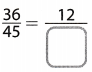First we use this rule
Apply fraction cross multiply: if $$\frac{a}{b}=\frac{c}{d}$$ then a ∙ d = b ∙ c
36x = 45 ∙ 12

36x = 540 (Multiply the numbers)
$$\frac{36 x}{36}=\frac{540}{36}$$ (Divide both sides by 5)
x = 15

The equivalent fraction becomes
$$\frac{36}{45}=\frac{12}{15}$$

Question 12.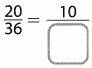First we use this rule
Apply fraction cross multiply: if $$\frac{a}{b}=\frac{c}{d}$$ then a ∙ d = b ∙ c
20x = 36 ∙ 10

20x = 360 (Multiply the numbers)
$$\frac{20 x}{20}=\frac{360}{20}$$ (Divide both sides by 20)
x = 18

The equivalent fraction becomes
$$\frac{20}{36}=\frac{10}{18}$$

List the first five multiples of each number.

Question 13.
3 ______________
List the first five multiples of 3

3 × 1 = 3
3 × 2 = 6 (Multiply 3 by the numbers 1, 2,)
3 × 3 = 9 (3, 4, and 5)
3 × 4 = 12
3 × 5 = 15

3, 6, 9, 12, 15

Question 14.
7 ______________
List the first five multiples of 7

7 × 1 = 7
7 × 2 = 14 (Multiply 7 by the numbers 1, 2,)
7 × 3 = 21 (3, 4 and 5)
7 × 4 = 28
7 × 5 = 35

7, 14, 21, 28, 35

Question 15.
8 ______________
List the first five multiples of 8
8 × 1 = 8
8 × 2 = 16 (Multiply 8 by the numbers 1, 2,)
8 × 3 = 24 (3, 4, and 5)
8 × 4 = 32
8 × 5 = 40

8, 16, 24, 32, 40

Visualize Vocabulary
Use the ✓ words to complete the graphic. Comparing Unit RatesUnderstand Vocabulary

Complete the sentences using the preview words.

Question 1.
A __________________ is a rate that compares two equivalent measurements.